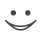# Get these flashcards, study & pass exams. For free! Even on iPhone/Android!

## Geometry Flashcards (27 Cards)

What postulate states if two lines intersect, then they intersect in exactly one point?
Postulate 1-2
What postulate states through any three noncollinear points there is exactly one plane?
Postulate 1-4
What postulate states if two planes intersect, then they intersect in exactly one line?
Postulate 1-3
What postulate states if three points A, B, and C are collinear and B is between A and C, then AB + BC = AC?
What postulate states if two figures are congruent, then their areas are equal?
Postulate 1-9
What law states if a conditional is true and its hypothesis is true, then its conclusion is true?
Law of Detachment
What law states if p -> q and q -> p are true statements, then p ---> r is a true statement?
Law of Syllogism
What theorem states vertical angles are congruent?
Theorem 2-1: Vertical Angles Theorem
What theorem states all right angles are congruent?
Theorem 2-4
What theorem states if two angles are congruent and supplementary, then each is a right angle?
Theorem 2-5
What theorem states if a transversal intersects two parallel lines, then alternate interior angles are congruent?
Theorem 3-1: Alternate Interior Angles Theorem
What theorem states if a transversal intersects two parallel lines, then same-side interior angles are supplementary?
Theorem 3-2: Same-Side Interior Angles Theorem
What theorem states if two lines are parallel to the same line, then they are parallel to each other?
Theorem 3-5
What postulate states if a transversal intersects two parallel lines, then corresponding angles are congruent?
Postulate 3-1: Corresponding Angles Postulate
What postulate states if two lines and a transversal form corresponding angles that are congruent, then the two lines are parallel?
Postulate 3-2: Converse of the Corresponding Angles Postulate
What postulate states  If three sides of one triangle are congruent to three sides of another triangle then the two triangles are congruent?
Postulate 4-1: Side Side Side (SSS) Postulate
What postulate states if two sides and the included angle of one triangle are congruent to two sides and the included angle of another triangle, then the two triangles are congruent?
Postulate 4-2: Side Angle Side (SAS) Postulate
What postulate states if two angles and an included side of one triangle are congruent to two angles and an included side of another triangle, then the two triangles are congruent?
Postulate 4-3: Angle Side Angle (ASA) Postulate
What theorem states If two sides of a triangle are congruent, then the angles opposite those sides are congruent?
Theorem 4-3: Isosceles Triangle Theorem
What theorem states the bisector of the vertex angle of an isosceles triangle is the perpendicular bisector of the base?
Theorem 4-5: Isosceles Vertex Bisector Theorem
What theorem states opposite sides of a parallelogram are congruent?
Theorem 6-1
What theorem states the diagonals of a parallelogram bisect each other?
Theorem 6-3
What theorem states each diagonal of a rhombus bisects two angles of the rhombus?
Theorem 6-9
What theorem states the diagonals of a rectangle are congruent?
Theorem 6-11
What theorem states the diagonals of a kite are perpendicular?
Theorem 6-17
What theorem states the diagonals of an isosceles trapezoid are congruent?
Theorem 6-16
What theorem states if both pairs of opposite sides of a quadrilateral are congruent, then the quadrilateral is a parallelogram?
Theorem 6-7Flashcard set info:
Author: CoboCards-User
Main topic: Mathematics
Topic: Geometry
Published: 12.01.2011

Card tags:
All cards (27)
no tagsReport abuse